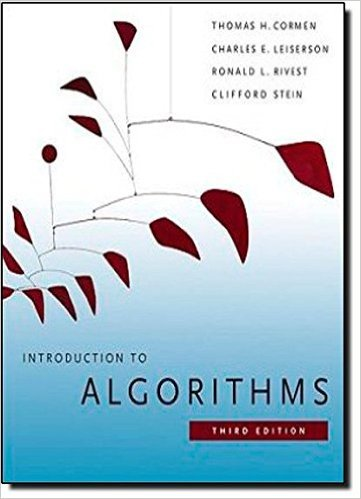×
Get Full Access to Introduction To Algorithms - 3 Edition - Chapter 33 - Problem 33-1
Get Full Access to Introduction To Algorithms - 3 Edition - Chapter 33 - Problem 33-1

×

# Convex layers Given a set Q of points in the plane, weISBN: 9780262033848 130

## Solution for problem 33-1 Chapter 33

Introduction to Algorithms | 3rd Edition

• Textbook Solutions
• 2901 Step-by-step solutions solved by professors and subject experts
• Get 24/7 help from StudySoup virtual teaching assistantsIntroduction to Algorithms | 3rd Edition

4 5 1 328 Reviews
10
2
Problem 33-1

Convex layers Given a set Q of points in the plane, we define the convex layers of Q inductively. The first convex layer of Q consists of those points in Q that are vertices of CH.Q/. For i>1, define Qi to consist of the points of Q with all points in convex layers 1; 2; : : : ; i 1 removed. Then, the ith convex layer of Q is CH.Qi/ if Qi ; and is undefined otherwise. a. Give an O.n2/-time algorithm to find the convex layers of a set of n points. b. Prove that .n lg n/ time is required to compute the convex layers of a set of n points with any model of computation that requires .n lg n/ time to sort n real numbers.

Step-by-Step Solution:
Step 1 of 3

Product Rule If F(x)=f(x)g(x), Then F’(x)=f(x)g’(x) + f’(x)g(x) Ex. d/dx [ (4x^3 - x^2 -1)(x^3 -2x^2 + 3x +1)] = (4x^3 - X^2 - 1) (3x^2- 4x + 3) = (12x^2 -2x)(x^3 - 2x^2 + 3x + 1) However the product rule is more useful for the product of different types of functions Ex. f(x)= x^2cos(x) g(x) = e^x arctan(x) Proof of the Product Rule Let F(x) = f(x)g(x) F’(x) = lim ((F(x + h ) - F(x))/h) h 0 =lim ((f(x + h )g( x + h) - f(x)g(x))/h) h 0 Subtract and add f(x + h)g(x) = lim ((f( x + h)g(x + h) - f(x + h)g(x) + f(x + h) - g(x) - f(x)g(x))/h) h 0 = lim ((f( x + h)(g( x + h) - g(x)) + g(x)(f( x + h) - f(x))/h) h 0 = lim ((f ( x+ h)(g( x + h) - g(x))/h) + (g(x)f(x + h) - f(x)/h) h 0 = lim (f( x + h) lim ((g(x + h) - g(x))/h

Step 2 of 3

Step 3 of 3

##### ISBN: 9780262033848

The full step-by-step solution to problem: 33-1 from chapter: 33 was answered by , our top Engineering and Tech solution expert on 11/10/17, 05:55PM. Introduction to Algorithms was written by and is associated to the ISBN: 9780262033848. Since the solution to 33-1 from 33 chapter was answered, more than 587 students have viewed the full step-by-step answer. This full solution covers the following key subjects: convex, points, layers, set, layer. This expansive textbook survival guide covers 35 chapters, and 151 solutions. This textbook survival guide was created for the textbook: Introduction to Algorithms, edition: 3. The answer to “Convex layers Given a set Q of points in the plane, we define the convex layers of Q inductively. The first convex layer of Q consists of those points in Q that are vertices of CH.Q/. For i>1, define Qi to consist of the points of Q with all points in convex layers 1; 2; : : : ; i 1 removed. Then, the ith convex layer of Q is CH.Qi/ if Qi ; and is undefined otherwise. a. Give an O.n2/-time algorithm to find the convex layers of a set of n points. b. Prove that .n lg n/ time is required to compute the convex layers of a set of n points with any model of computation that requires .n lg n/ time to sort n real numbers.” is broken down into a number of easy to follow steps, and 130 words.

Unlock Textbook Solution